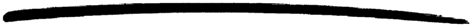# fixed

fixed

### MODULE SUMMARY

the corba fixed type

### DESCRIPTION

This module contains functions that gives an interface to the CORBA fixed type.

The type Fixed used below is defined as:

```      -record(fixed, {digits, scale, value}).

```

where digits is the total amount of digits it consists of and scale is the number of fractional digits. The value field contains the actual Fixed value represented as an integer. The limitations of each field are:

• Digits - integer(), -1 > Digits < 32
• Scale - integer(), -1 > Scale =< Digits
• Value - integer(), range (31 digits): ±9999999999999999999999999999999

Since the Value part is represented by an integer, it is vital that the Digits and Scale values are correct. This also means that trailing zeros cannot be left out in some cases:

• fixed<5,3> eq. 03.140d eq. 3140
• fixed<3,2> eq. 3.14d eq. 314

Leading zeros can be left out.

For your convenience, this module exports functions which handle unary (-) and binary (+-*/) operations legal for the Fixed type. Since a unary + have no effect, this module do not export such a function. Any of the binary operations may cause an overflow (i.e. more than 31 significant digits; leading and trailing zeros are not considered significant). If this is the case, the Digit and Scale values are adjusted and the Value truncated (no rounding performed). This behavior is compliant with the OMG CORBA specification. Each binary operation have the following upper bounds:

• Fixed1 + Fixed2 - fixed<max(d1-s1,d2-s2) + max(s1,s2) + 1, max(s1,s2)>
• Fixed1 - Fixed2 - fixed<max(d1-s1,d2-s2) + max(s1,s2) + 1, max(s1,s2)>
• Fixed1 * Fixed2 - fixed<d1+d2, s1+s2>
• Fixed1 / Fixed2 - fixed<(d1-s1+s2) + Sinf ,Sinf >

A quotient may have an arbitrary number of decimal places, which is denoted by a scale of Sinf.

### EXPORTS

Types:

Result = Fixed Type | {'EXCEPTION', #'BAD_PARAM'{}}

This function creates a new instance of a Fixed Type. If the limitations is not fulfilled (e.g. overflow) an exception is raised.

Types:

Result = TypeCode | {'EXCEPTION', #'BAD_PARAM'{}}

Returns the TypeCode which represents the supplied Fixed type. If the parameter is not of the correct type, an exception is raised.

Types:

Result = Fixed1 + Fixed2 | {'EXCEPTION', #'BAD_PARAM'{}}

Performs a Fixed type addition. If the parameters are not of the correct type, an exception is raised.

Types:

Result = Fixed1 - Fixed2 | {'EXCEPTION', #'BAD_PARAM'{}}

Performs a Fixed type subtraction. If the parameters are not of the correct type, an exception is raised.

Types:

Result = Fixed1 * Fixed2 | {'EXCEPTION', #'BAD_PARAM'{}}

Performs a Fixed type multiplication. If the parameters are not of the correct type, an exception is raised.

Types:

Result = Fixed1 / Fixed2 | {'EXCEPTION', #'BAD_PARAM'{}}

Performs a Fixed type division. If the parameters are not of the correct type, an exception is raised.

Types:

Result = -Fixed | {'EXCEPTION', #'BAD_PARAM'{}}

Negates the supplied Fixed type. If the parameter is not of the correct type, an exception is raised.

orber 3.6.13